## Exercises

1. Consider the planning problem shown in Figure 2.21. Let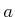be the initial state, and let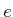be the goal state.
1. [(a)] Use backward value iteration to determine the stationary cost-to-go.
2. [(b)] Do the same but instead use forward value iteration.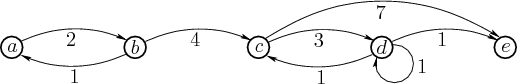2. Try to construct a worst-case example for best-first search that has properties similar to that shown in Figure 2.5, but instead involves moving in a 2D world with obstacles, as introduced in Example 2.1.

3. It turns out that value iteration can be generalized to a cost functional of the form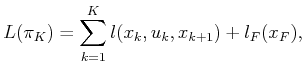(2.41)

in which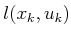in (2.4) has been replaced by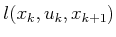.
1. Show that the dynamic programming principle can be applied in this more general setting to obtain forward and backward value iteration methods that solve the fixed-length optimal planning problem.
2. Do the same but for the more general problem of variable-length plans, which uses termination conditions.
4. The cost functional can be generalized to being stage-dependent, which means that the cost might depend on the particular stagein addition to the state,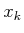and the action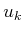. Extend the forward and backward value iteration methods of Section 2.3.1 to work for this case, and show that they give optimal solutions. Each term of the more general cost functional should be denoted as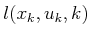.
5. Recall from Section 2.3.2 the method of defining a termination action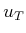to make the value iterations work correctly for variable-length planning. Instead of requiring that one remains at the same state, it is also possible to formulate the problem by creating a special state, called the terminal state,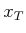. Wheneveris applied, the state becomes. Describe in detail how to modify the cost functional, state transition equation, and any other necessary components so that the value iterations correctly compute shortest plans.
6. Dijkstra's algorithm was presented as a kind of forward search in Section 2.2.1.
1. Develop a backward version of Dijkstra's algorithm that starts from the goal. Show that it always yields optimal plans.
2. Describe the relationship between the algorithm from part (a) and the backward value iterations from Section 2.3.2.
3. Derive a backward version of the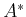algorithm and show that it yields optimal plans.
7. Reformulate the general forward search algorithm of Section 2.2.1 so that it is expressed in terms of the STRIPS-like representation. Carefully consider what needs to be explicitly constructed by a planning algorithm and what is considered only implicitly.
8. Rather than using bit strings, develop a set-based formulation of the logic-based planning problem. A state in this case can be expressed as a set of positive literals.
9. Extend Formulation 2.4 to allow disjunctive goal sets (there are alternative sets of literals that must be satisfied). How does this affect the binary string representation?
10. Make a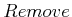operator for Example 2.17 that takes a battery away from the flashlight. For this operator to apply, the battery must be in the flashlight and must not be blocked by another battery. Extend the model to allow enough information for theoperator to function properly.
11. Model the operation of the sliding-tile puzzle in Figure 1.1b using the STRIPS-like representation. You may use variables in the operator definitions.
12. Find the complete set of plans that are implicitly encoded by Example 2.7.
13. Explain why, in Formulation 2.4,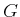needs to include both positive and negative literals, whereas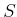only needs positive literals. As an alternative definition, couldhave contained only negative literals? Explain.
14. Using Formulation 2.4, model a problem in which a robot checks to determine whether a room is dark, moves to a light switch, and flips on the light. Predicates should indicate whether the robot is at the light switch and whether the light is on. Operators that move the robot and flip the switch are needed.
15. Construct a planning graph for the model developed in Exercise 14.
16. Express the model in Exercise 14 as a Boolean satisfiability problem.
17. In the worst case, how many terms are needed for the Boolean expression for planning as satisfiability? Express your answer in terms of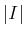,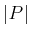,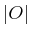,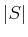, and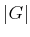.

Implementations

18. Usingsearch, the performance degrades substantially when there are many alternative solutions that are all optimal, or at least close to optimal. Implementsearch and evaluate it on various grid-based problems, based on Example 2.1. Compare the performance for two different cases:
1. Using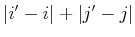as the heuristic, as suggested in Section 2.2.2.
2. Using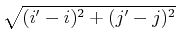as the heuristic.
19. Implement, breadth-first, and best-first search for grid-based problems. For each search algorithm, design and demonstrate examples for which one is clearly better than the other two.
21. Try to improve the method used to solve Exercise 18 by detecting when the search might be caught in a local minimum and performing random walks to try to escape. Try using best-first search instead of. There is great flexibility in possible approaches. Can you obtain better performance on average for any particular examples?